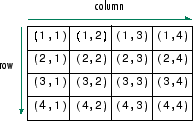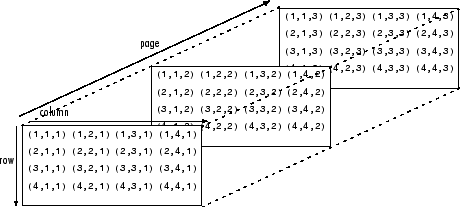# 多维数组

MATLAB® 中的多维数组是指具有两个以上维度的数组。在矩阵中，两个维度由行和列表示。### 创建多维数组

`A = [1 2 3; 4 5 6; 7 8 9]`
```A = 3×3 1 2 3 4 5 6 7 8 9 ```

`A(:,:,2) = [10 11 12; 13 14 15; 16 17 18]`
```A = A(:,:,1) = 1 2 3 4 5 6 7 8 9 A(:,:,2) = 10 11 12 13 14 15 16 17 18 ```

`cat` 函数可用于构造多维数组。例如，在 `A` 后以串联方式添加第三页，由此创建一个新的三维数组 `B`。第一个参数指示要沿哪一个维度进行串联。

`B = cat(3,A,[3 2 1; 0 9 8; 5 3 7])`
```B = B(:,:,1) = 1 2 3 4 5 6 7 8 9 B(:,:,2) = 10 11 12 13 14 15 16 17 18 B(:,:,3) = 3 2 1 0 9 8 5 3 7 ```

`B(:,:,4) = 0`
```B = B(:,:,1) = 1 2 3 4 5 6 7 8 9 B(:,:,2) = 10 11 12 13 14 15 16 17 18 B(:,:,3) = 3 2 1 0 9 8 5 3 7 B(:,:,4) = 0 0 0 0 0 0 0 0 0 ```

### 访问元素

`A`
```A = A(:,:,1) = 1 2 3 4 5 6 7 8 9 A(:,:,2) = 10 11 12 13 14 15 16 17 18 ```
`elA = A(1,2,2)`
```elA = 11 ```

`C = A(:,[1 3],:)`
```C = C(:,:,1) = 1 3 4 6 7 9 C(:,:,2) = 10 12 13 15 16 18 ```

`D = A(2:3,:,:)`
```D = D(:,:,1) = 4 5 6 7 8 9 D(:,:,2) = 13 14 15 16 17 18 ```

### 操作数组```A = [1 2 3 4 5; 9 0 6 3 7; 8 1 5 0 2]; A(:,:,2) = [9 7 8 5 2; 3 5 8 5 1; 6 9 4 3 3]; B = reshape(A,[6 5])```
```B = 6×5 1 3 5 7 5 9 6 7 5 5 8 5 2 9 3 2 4 9 8 2 0 3 3 8 1 1 0 6 4 3 ```

`reshape` 逐列操作，沿 `A` 中各列连续逐一提取元素来创建新矩阵，从第一页开始，之后是第二页。

```M(:,:,1) = [1 2 3; 4 5 6; 7 8 9]; M(:,:,2) = [0 5 4; 2 7 6; 9 3 1]```
```M = M(:,:,1) = 1 2 3 4 5 6 7 8 9 M(:,:,2) = 0 5 4 2 7 6 9 3 1 ```

`P1 = permute(M,[2 1 3])`
```P1 = P1(:,:,1) = 1 4 7 2 5 8 3 6 9 P1(:,:,2) = 0 2 9 5 7 3 4 6 1 ```

`P2 = permute(M,[3 2 1])`
```P2 = P2(:,:,1) = 1 2 3 0 5 4 P2(:,:,2) = 4 5 6 2 7 6 P2(:,:,3) = 7 8 9 9 3 1 ```

`A = repmat(5,[2 3 1 4])`
```A = A(:,:,1,1) = 5 5 5 5 5 5 A(:,:,1,2) = 5 5 5 5 5 5 A(:,:,1,3) = 5 5 5 5 5 5 A(:,:,1,4) = 5 5 5 5 5 5 ```
`szA = size(A)`
```szA = 1×4 2 3 1 4 ```
`numdimsA = ndims(A)`
```numdimsA = 4 ```

`B = squeeze(A)`
```B = B(:,:,1) = 5 5 5 5 5 5 B(:,:,2) = 5 5 5 5 5 5 B(:,:,3) = 5 5 5 5 5 5 B(:,:,4) = 5 5 5 5 5 5 ```
`szB = size(B)`
```szB = 1×3 2 3 4 ```
`numdimsB = ndims(B)`
```numdimsB = 3 ```